Michael Pidwirny

# LEARNING GOALS

The primary purpose of this laboratory is to see how temporal and spatial geometric relationships between the Earth and the Sun influence the amount of solar radiation (insolation) received at the Earth’s surface. This radiation is the primary source of energy for processes like the heating of the atmosphere, the development of atmospheric circulation, and the production of organic molecules via plant photosynthesis.

Upon completion of this laboratory you will be able to:

1. Understand how the orbital relationships between the Sun and the Earth produce changes in day length and height of the Sun in our sky for the various locations on our planet.
2. Determine a location’s solar angle for particular hour of day and for any day of the year.
3. Calculate the relationship between Sun angle and the intensity of incoming sunlight.
4. Comprehend how the orbital relationships between the Sun and the Earth influence the flow of the Sun’s insolation to the Earth.

# INSOLATION

(incoming solar radiation) can be defined as the solar radiation received in a specified period on a unit area on or above the Earth’s surface. The quantity insolation present at any location on the Earth is dependent on:

• date and time,
• latitude (involving variations of length of day and degree of obliquity of the Sun’s rays),
• intensity of solar output,
• slope and aspect of the location’s surface,
• distance of the Earth from the Sun, and
• degree of transparency of the atmosphere.

Insolation is the main source of energy for many atmospheric processes. Sunlight heats the air around us, provides the energy for the evaporation of water and melting of ice, and plays and important role in the formation of wind and large-scale air circulation. Sunlight also provides the energy for plant photosynthesis.

All of us are aware of the fact that the quantity of sunlight varies over time and space. Over a 24-hour period, we know that sunlight is generally strongest around noon hour and nonexistent during the time of day we call night. Many locations on our planet experience yearly variations in the quantity of insolation. If these variations are large enough, they can cause the annual march of the seasons. Across the dimension of space, most of us are familiar with the fact that locations near the Equator experience summer like conditions are year round. Near the Equator, places receive high quantities of insolation all year long. Locations near the poles only receive significant amounts of insolation during a relatively short summer period. For this reason, these localities have cold winter conditions during most of the year.

# EARTH ROTATION AND REVOLUTION

The term refers to the spinning of the Earth on its axis (Figure 1.1). One rotation takes exactly twenty-four hours and is called a . If you could look down at the Earth’s North Pole from space you would notice that the direction of rotation is counter-clockwise. The opposite is true if you viewed the Earth from the South Pole. One of the most obvious effects of Earth rotation is the daily movement of the Sun across the sky. Of course, the Sun is not the object doing the movement in this occurrence. The perceived movement of the Sun is actually caused by the fact that the Earth is spinning on its axis in space relative to a stationary Sun.

The annual orbit of the Earth around the Sun is called . This celestial motion takes 365 1/4 days to complete one cycle. Further, the Earth’s orbit around the Sun is not circular, but elliptical (Figure 1.2). An elliptical orbit causes the Earth’s distance from the Sun to vary annually. However, this phenomenon does not cause the seasons! This annual variation in the distance from the Sun does influence the amount of solar radiation intercepted by the Earth by approximately 6%. On January 3rd, , the Earth is closest to the Sun (147.3 million km). The Earth is farthest from the Sun on July 4th, or (152.1 million km). The average distance of the Earth from the Sun over a one-year period is about 150 million km.

Figure 1.1. YouTube video showing the movement of the Earth about its axis is known as Earth rotation. The direction of this movement varies with the viewer’s position. From the North Pole the rotation appears to move in a counter-clockwise fashion. Looking down at the South Pole the Earth’s rotation appears clockwise.

## TILT OF THE EARTH’S AXIS

The Earth’s axis is not perpendicular to the , but inclined at a fixed angle of 23.5°. Further, the northern end of the Earth’s axis always points to the same place in space (North Star). Note that the angle of the Earth’s axis in relation to the plane of the ecliptic remains unchanged. However, the relative position of the Earth’s axis to the Sun does change during this cycle (Figures 1.3, 1.4, and 1.5). This circumstance is responsible for the annual changes in the relative height of the Sun above the horizon. It also causes the seasons, by controlling the intensity and duration of sunlight received by locations on the Earth.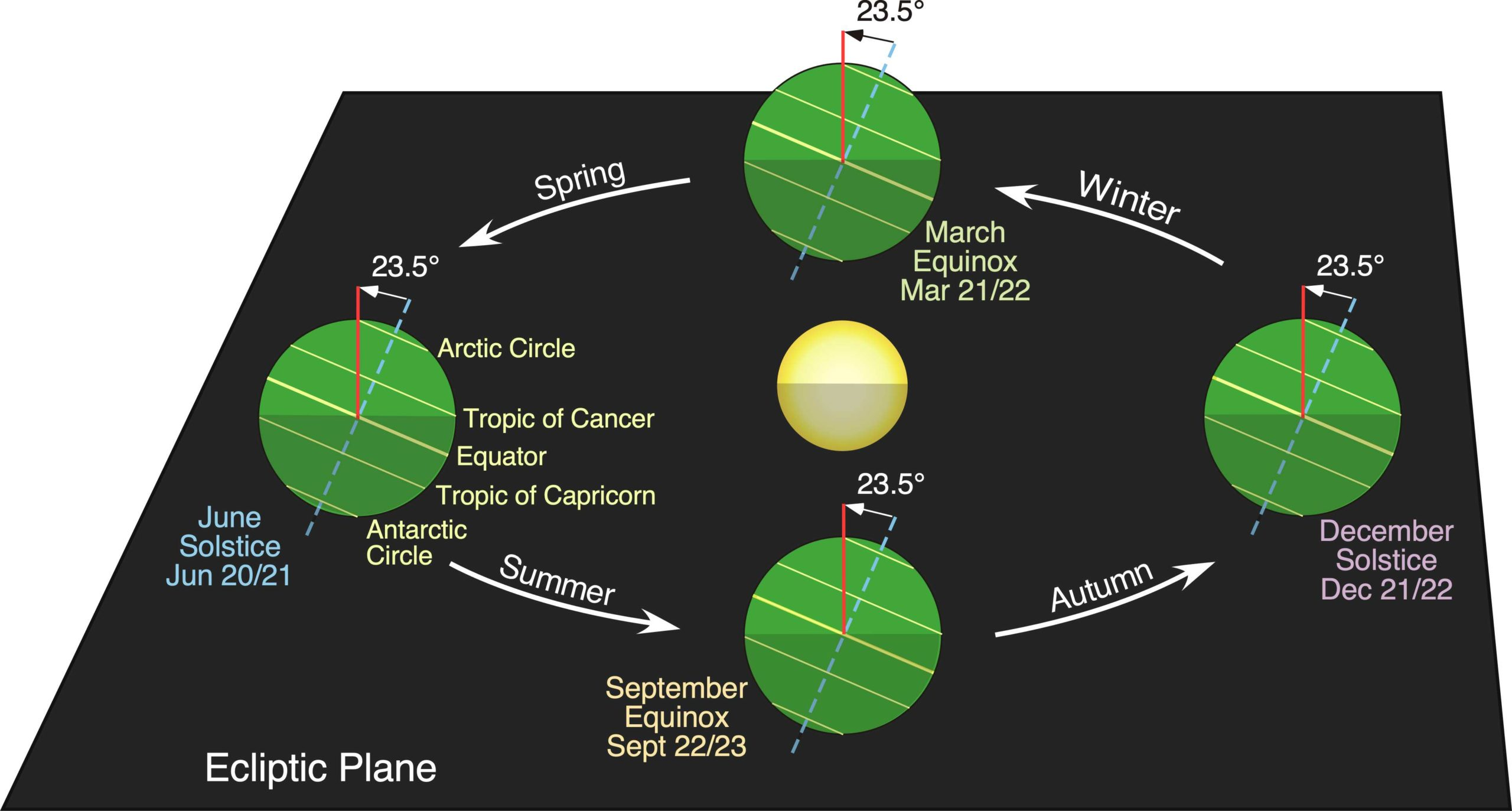Figure 1.3. Geometric relationships between the Earth and the Sun as seen from above the North Pole. Note that only a portion of the Earth is illuminated by the Sun and this portion changes with Earth rotation and revolution. The line separating the illuminated and non-illuminated parts of our planet is known as the circle of illumination. Not drawn to scale. Image Copyright: Michael Pidwirny.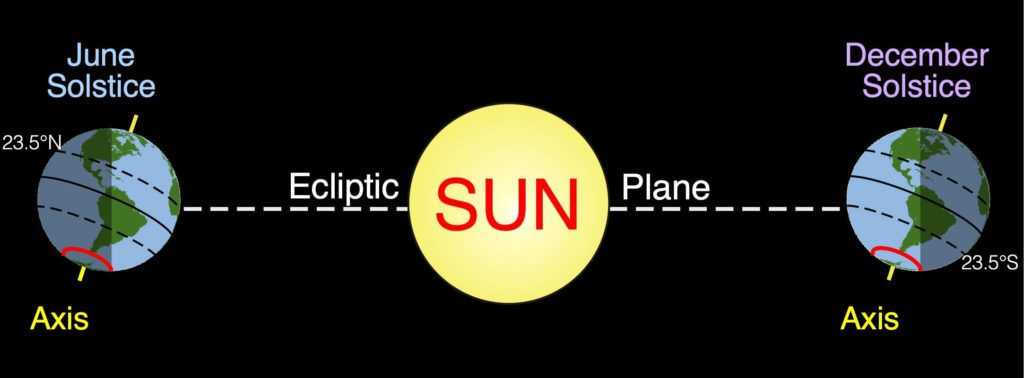Figure 1.4. During the June Solstice, the Earth’s North Pole is tilted 23.5° towards the Sun relative to the circle of illumination. This phenomenon keeps all places above a latitude of 66.5° N in 24 hours of sunlight, while locations below a latitude of 66.5° S are in darkness. The North Pole is tilted 23.5° away from the Sun relative to the circle of illumination during the December Solstice. On this date, all places above a latitude of 66.5° N are now in darkness, while locations below a latitude of 66.5° S receive 24 hours of daylight. The white semi-circles shown in the graphic are the Equator and Antarctic Circle. Not drawn to scale. Image Copyright: Michael Pidwirny.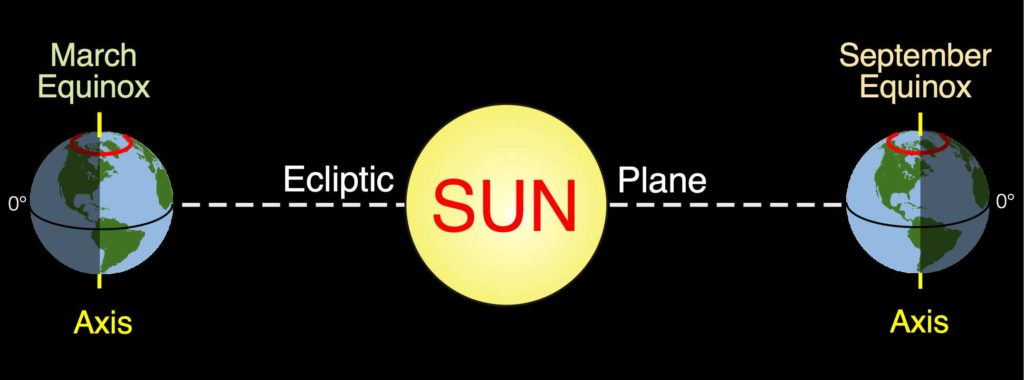Figure 1.5. During the equinoxes, the axis of the Earth is not tilted toward or away from the Sun, and the circle of illumination cuts through the poles. This situation does not suggest that the 23.5° tilt of the Earth no longer exists. The vantage point of this graphic shows that the Earth’s axis is inclined 23.5° toward the viewer for both dates (see Figure 1.3). The white semi-circles shown in the graphic are the Equator and Arctic Circle. Not drawn to scale. Image Copyright: Michael Pidwirny.

On June 21 or 22, the , the Earth is positioned in its orbit so that the is leaning 23.5° toward the Sun (Figures 1.3 and 1.4). During the Summer Solstice, all locations north of the Equator have day lengths greater than twelve hours, while all locations South of the Equator have day lengths less than twelve hours (see Table 1.2 below). On December 21 or 22, the , the Earth is positioned so that the is leaning 23.5° toward the Sun (Figures 1.3 and 1.4). During the Winter Solstice, all locations North of the Equator have day lengths less than twelve hours, while all locations South of the Equator have day lengths greater than twelve hours (see Table 1.3 below).

On September 22 or 23, the , neither pole is tilted toward the Sun (Figures 1.3 and 1.5). March 20 or 21 marks the arrival of the when once again the poles are not tilted toward the Sun. Day lengths on both of these days, regardless of latitude, are exactly 12 hours.

The following YouTube animation in Figure 1.6 summarizes the information covered so far, please watch it.

Figure 1.6. YouTube animation explaining how the tilt of the Earth influences Sun angles and day lengths for the days associated with the solstices and equinoxes.

# ANGLE OF INCIDENCE

Yearly changes in the relative position of the Earth’s axis cause the location of the Sun to wander 47° across our skies. Changes in the location of the Sun in the sky have a direct effect on the intensity of solar radiation. The intensity of solar radiation received on the Earth’s surface is largely a function of the , the angle at which the Sun’s rays strike the ground. If the Sun is positioned directly overhead or 90° from the horizon, the incoming insolation strikes the surface of the Earth at right angles and is most intense. If the Sun is 45° above the horizon, the incoming insolation strikes the Earth’s surface at an angle. This causes the rays to be spread out over a larger surface area reducing the intensity of the radiation. Figure 1.7 models the effect of changing the angle of incidence from 90° to 45°. As illustrated, the lower Sun angle (45°) causes the radiation to be received over a much larger surface area. This surface area is approximately 40% greater than the area covered by an angle of 90°. The lower angle also reduces the intensity of the incoming rays by 30%.

We can also model the effect the angle of incidence has on insolation intensity with the following simple equation:

## Intensity = SIN (A)

where, A is the angle of incidence and SIN is the sine function found on most calculators. Using this equation, we can determine that an angle of 90° gives us a value of 1.00 or 100% (1.00 x 100). Let us compare this maximum value with values determined for other angles of incidence.

sin 80 = 0.98 or 98%

sin 70 = 0.94 or 94%

sin 60 = 0.87 or 87%

sin 50 = 0.77 or 77%

sin 40 = 0.64 or 64%

sin 30 = 0.50 or 50%

sin 20 = 0.34 or 34%

sin 10 = 0.17 or 17%

sin 0 = 0.00 or 0%

# ANNUAL CHANGES IN DAY LENGTH

The yearly changes in the position of the Earth’s axis relative to the plane of the ecliptic also causes seasonal variations in day length to all locations outside of the Equator. Longest days occur during the summer solstice for locations north of the Equator and on the winter solstice for locations in the Southern Hemisphere. The Equator experiences equal day and night on every day of the year. Day and night is also of equal length for all Earth locations on the autumnal and vernal equinoxes. Figure 1.8 describes the change in the length of day for locations at the Equator, 10°, 30°, 50°, 60°, and 70° North over a one-year period. The illustration suggests that days are longer than nights in the Northern Hemisphere from the March Equinox to the September Equinox. Between the September Equinox to March Equinox days are shorter than nights in the Northern Hemisphere. The opposite is true in the Southern Hemisphere. The graph also shows that the seasonal (winter to summer) variation in day length increases with increasing latitude.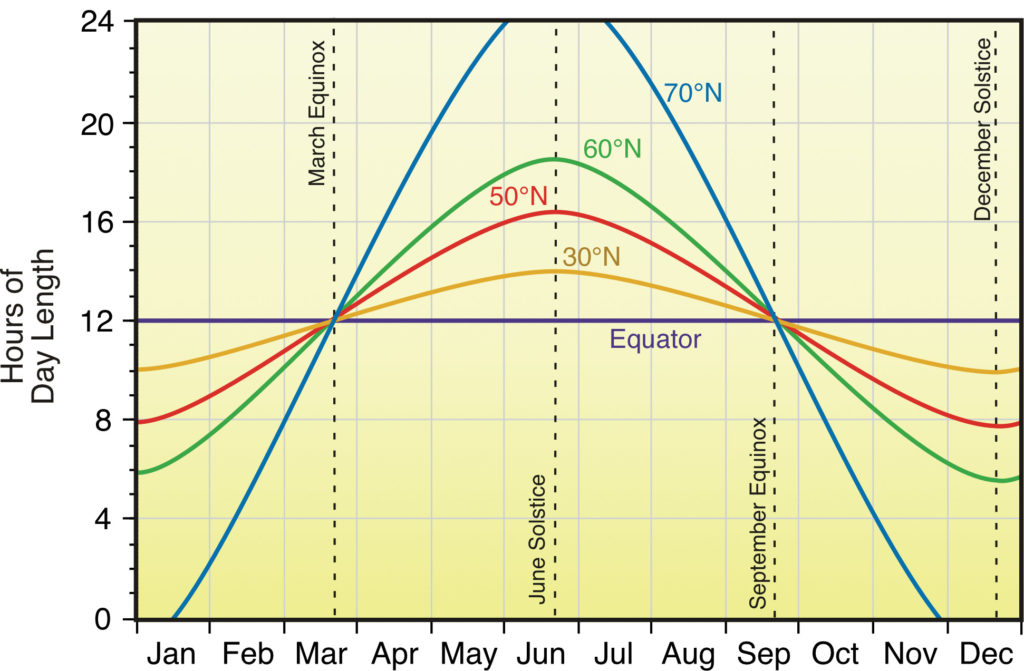Figure 1.8. Annual variations in day length for locations at the Equator, 30°, 50°, 60°, and 70° North latitude. Image Copyright: Michael Pidwirny.

# QUESTION 1

##### For a location at 50° North examine the graph below and answer the following questions: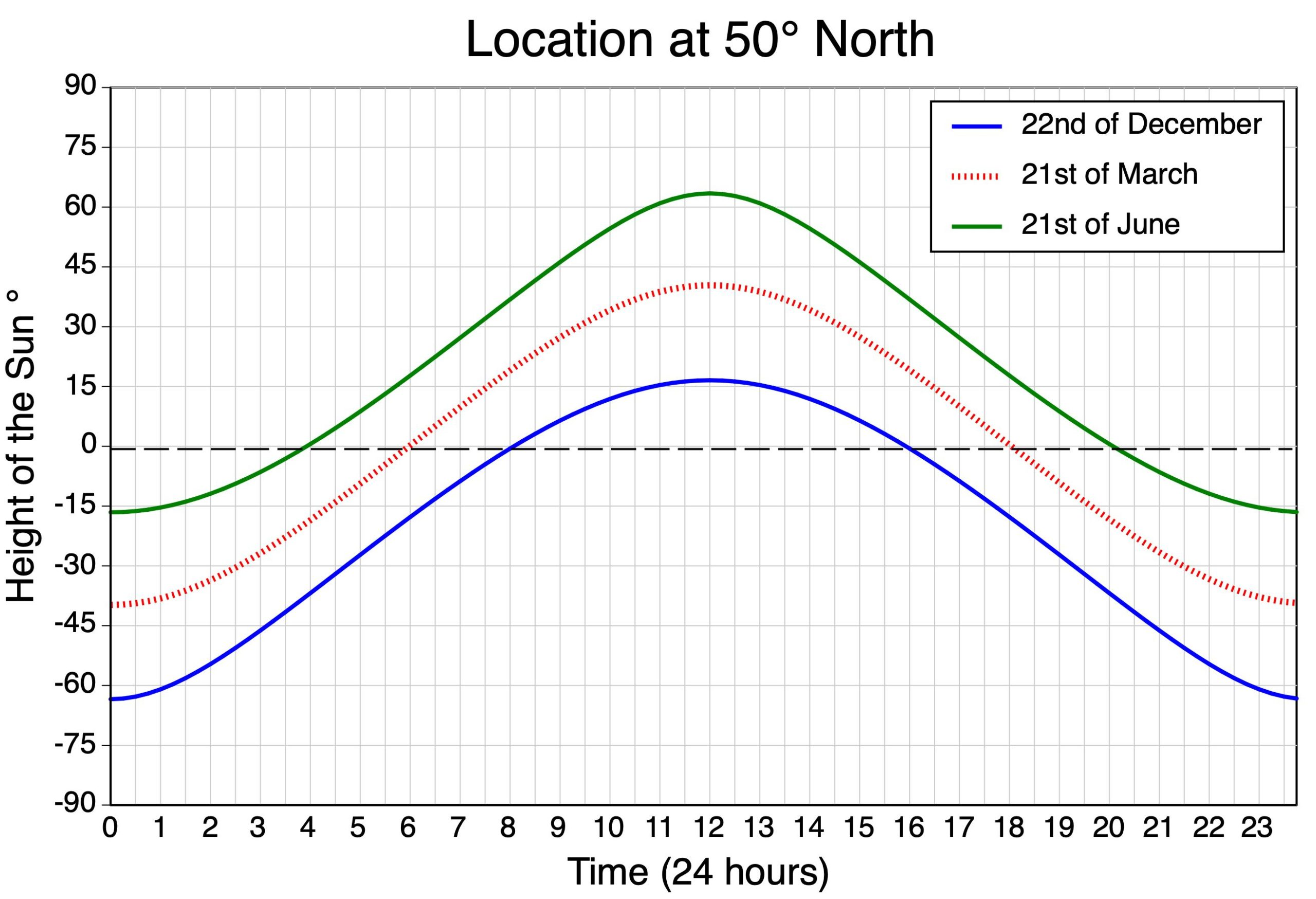Figure 1.9. Height of the Sun above (+) or below (-) the horizon at different times during the day for December 22, March 21, and June 21 for a location found at 50° North. Image Copyright: Michael Pidwirny.
##### For the date December 22 () –

1.1) At what time does the Sun first appear above the horizon (sunrise)?

8:09 AM.

6:00 AM.

3:51 AM.

1.2) At what time does the Sun have the greatest altitude?

11:45 AM.

12:00 AM (noon).

12:15 PM.

1.3) At what time does the Sun first appear below the horizon (sunset)?

8:09 PM.

6:00 PM.

3:51 PM.

1.4) On December 22, the North Pole is

tilted 23.5 degrees towards the Sun.

tilted 23.5 degrees away from the Sun.

not tilted towards or away from the Sun (perpendicular).

##### For the date March 21 () –

1.5) At what time does the Sun first appear above the horizon (sunrise)?

8:09 AM.

6:00 AM.

3:51 AM.

1.6) At what time does the Sun have the greatest altitude?

11:45 AM.

12:00 AM (noon).

12:15 PM.

1.7) At what time does the Sun first appear below the horizon (sunset)?

8:09 PM.

6:00 PM.

3:51 PM.

1.8) On March 21, the North Pole is

tilted 23.5 degrees towards the Sun.

B tilted 23.5 degrees away from the Sun.

not tilted towards or away from the Sun (perpendicular).

##### For the date June 21 () –

1.9) At what time does the Sun first appear above the horizon (sunrise)?

8:09 AM.

6:00 AM.

3:51 AM.

1.10) At what time does the Sun have the greatest altitude?

11:45 AM.

12:00 AM (noon).

12:15 PM.

1.11) At what time does the Sun first appear below the horizon (sunset)?

8:09 PM.

6:00 PM.

3:51 PM.

1.12) On March 21, the North Pole is

tilted 23.5 degrees towards the Sun.

tilted 23.5 degrees away from the Sun.

not tilted towards or away from the Sun (perpendicular).

1.13) On all of the dates above, the difference in the maximum height of the Sun between the June Solstice and December Solstice for 50° North is?

23.5 degrees.

47 degrees.

0 degrees.

# QUESTION 2

For a location at 75° South examine the graph below and answer the following questions: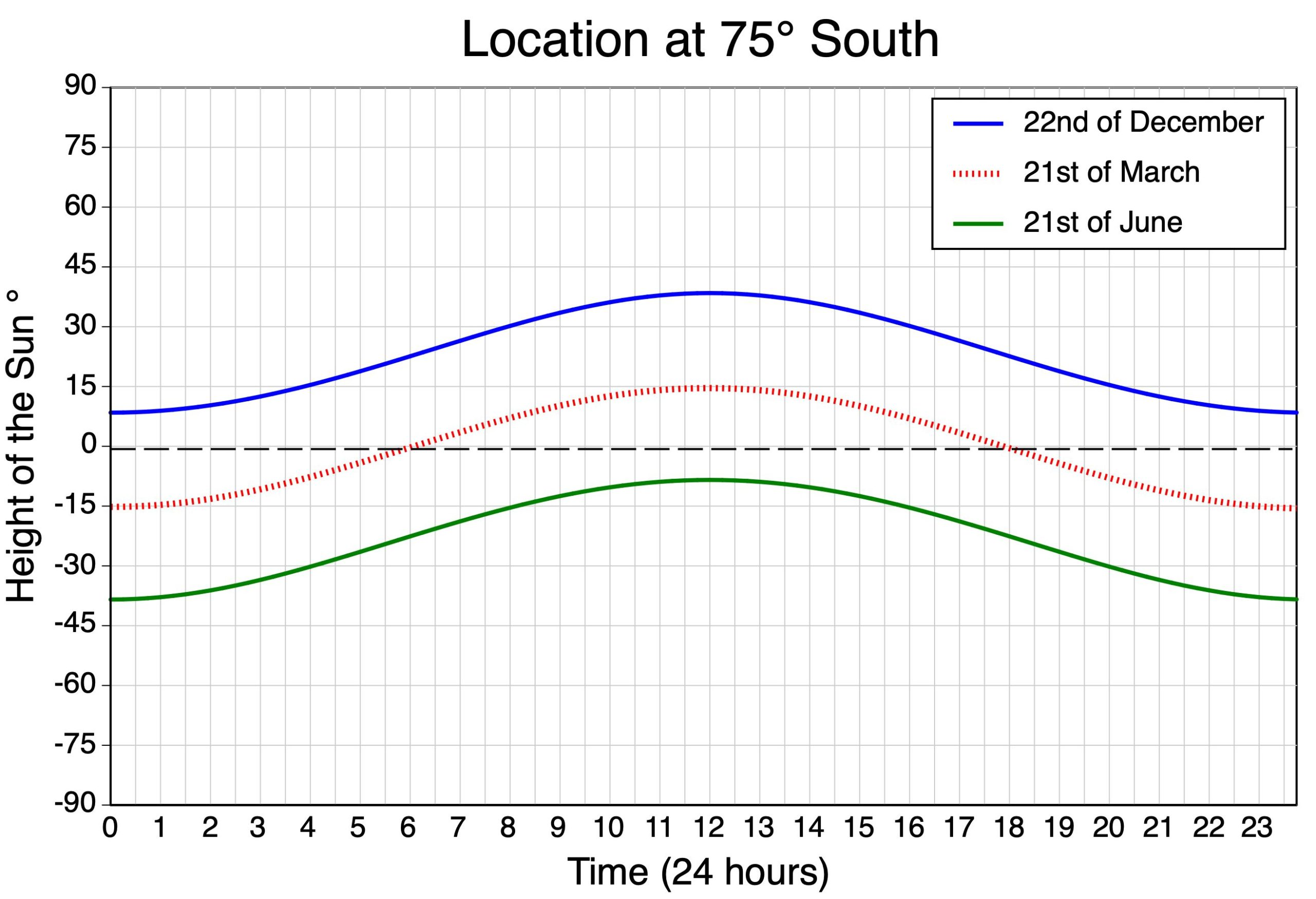Figure 1.10. Height of the Sun above (+) or below (-) the horizon at different times during the day for December 22, March 21, and June 21 for a location found at 75° South. Image Copyright: Michael Pidwirny.

For the date December 22 (December Solstice) –

2.1) At what time does the Sun first appear above the horizon (sunrise)?

The Sun is always above the horizon – 24 hours of day light.

6:00 AM.

The Sun is always below the horizon – 24 hours of night.

2.2) At what time does the Sun have the greatest altitude?

6:00 AM.

12:00 AM (noon).

6:00 PM.

2.3) On December 22, the South Pole is

tilted 23.5 degrees towards the Sun.

tilted 23.5 degrees away from the Sun.

not tilted towards or away from the Sun (perpendicular).

For the date March 21 (March Equinox) –

2.4) At what time does the Sun first appear above the horizon (sunrise)?

The Sun is always above the horizon – 24 hours of day light.

6:00 AM.

The Sun is always below the horizon – 24 hours of night.

2.5) At what time does the Sun have the greatest altitude?

11:45 AM.

12:00 AM (noon).

12:15 PM.

2.6) At what time does the Sun first appear below the horizon (sunset)?

7:00 PM.

6:00 PM.

5:00 PM.

2.7) On March 21, the North Pole is

tilted 23.5 degrees towards the Sun.

tilted 23.5 degrees away from the Sun.

not tilted towards or away from the Sun (perpendicular).

For the date June 21 (June Solstice) –

2.8) At what time does the Sun first appear above the horizon (sunrise)?

The Sun is always above the horizon – 24 hours of day light.

6:00 AM.

The Sun is always below the horizon – 24 hours of night.

2.9) At what time does the Sun have the greatest altitude?

11:45 AM.

12:00 AM (noon) but its below the horizon.

12:15 PM.

2.10) On June 21, the North Pole is

tilted 23.5 degrees towards the Sun.

tilted 23.5 degrees away from the Sun.

not tilted towards or away from the Sun (perpendicular).

2.11) On all of the dates above, the difference in the maximum height of the Sun between the June Solstice and December Solstice for 75° South is?

23.5 degrees.

47 degrees.

0 degrees.

# QUESTION 3

For a location at 5° South examine the graph below and answer the following questions: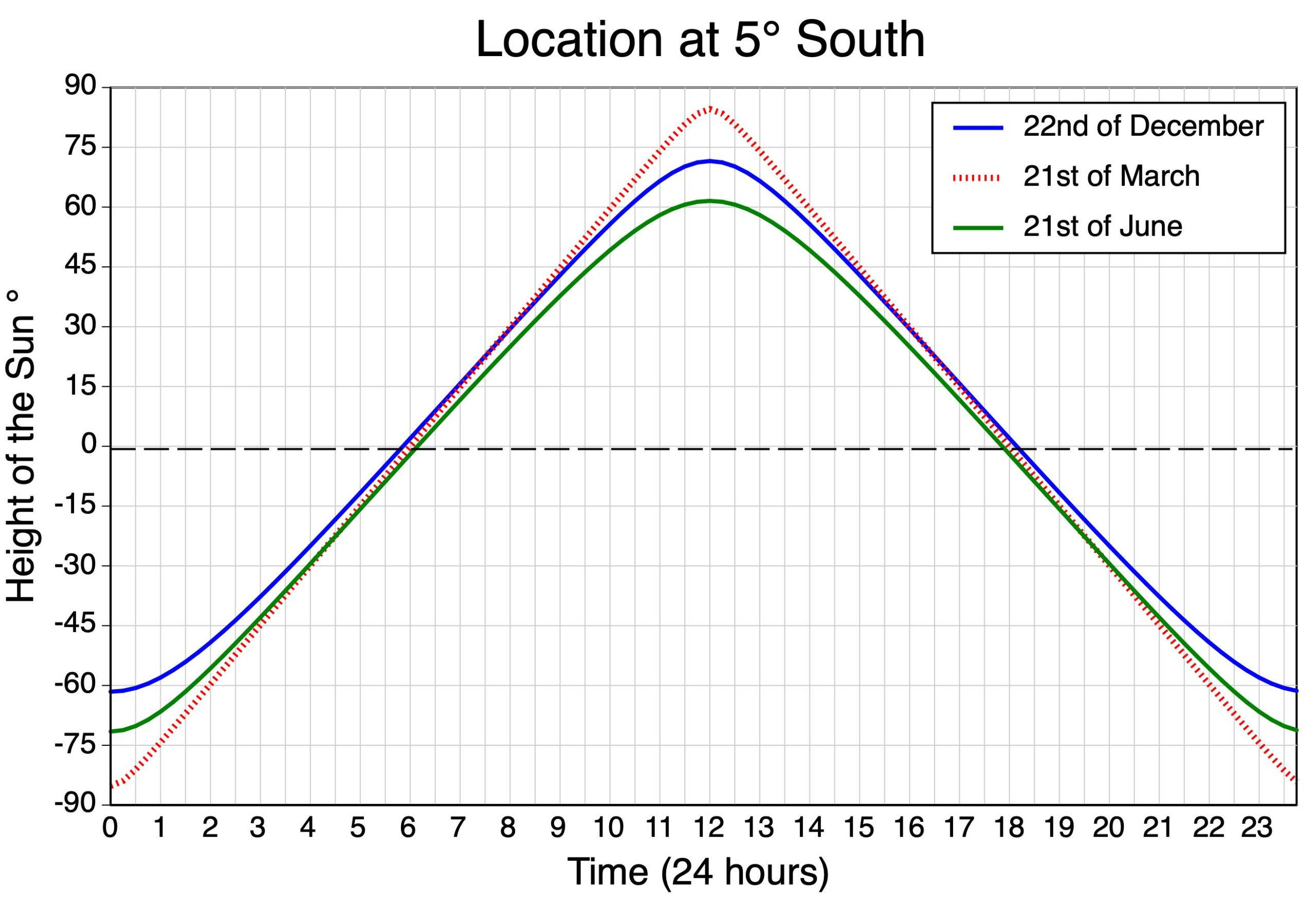Figure 1.11. Height of the Sun above (+) or below (-) the horizon at different times during the day for December 22, March 21, and June 21 for a location found at 5° South. Image Copyright: Michael Pidwirny.

For the date December 22 (December Solstice) –

3.1) At what time does the Sun first appear above the horizon (sunrise)?

5:45 AM.

6:00 AM.

6:15 AM.

3.2) At what time does the Sun have the greatest altitude?

11:45 AM.

12:00 AM (noon).

12:15 PM.

3.3) At 5° South, on December 22 day length is

about 12 hours and 30 minutes long.

exactly 12 hours long.

about 11 hours and 30 minutes long.

For the date March 21 (March Equinox) –

3.4) At 5° South, on March 21 day length is

about 12 hours and 30 minutes long.

exactly 12 hours long.

about 11 hours and 30 minutes long.

3.5) At what time does the Sun have the greatest altitude?

11:45 AM.

12:00 AM (noon).

12:15 PM.

3.6) At what time does the Sun first appear below the horizon (sunset)?

5:45 PM.

6:00 PM.

6:15 PM.

3.7) On March 21, the North Pole is

tilted 23.5 degrees towards the Sun.

tilted 23.5 degrees away from the Sun.

not tilted towards or away from the Sun (perpendicular).

For the date June 21 (June Solstice) –

3.8) At 5° South, on June 22 day length is

about 12 hours and 30 minutes long.

exactly 12 hours long.

about 11 hours and 30 minutes long.

3.9) At what time does the Sun first appear above the horizon (sunrise)?

5:45 AM.

6:00 AM.

6:15 AM.

# QUESTION 4

Solar noon can be defined as the time during the day when the Sun is aligned with True North and True South Solar noon also represent the time when the Sun reaches its maximum altitude (which is usually measured as an angle) above the horizon. The following equation can be used to derive the Sun angle at solar noon:

## θ = 90° –φ± δ

where θ  (theta) is the noon Sun angle above the horizon, φ  (phi) is the latitude, and δ  (delta) is the solar declination; all values are in degrees.

This equation gives you the Sun angle when looking towards the Equator at noon. In tropical latitudes (between 23½° N and 23½° S), values of q greater than 90° may occur for some dates. When this occurs, the noonday Sun is actually “behind you” when looking towards the Equator. Under these circumstances, θ  should be recalculated as:

## θ = 180 – (90° –φ± δ)

Note: when using the equations above, treat all values of δ  as positive when the location is tilted toward the Sun (summer half of the year), and negative when the location is tilted away from the Sun (winter half of the year).

The following YouTube video has some more interesting information regarding solar noon, please watch it:

Figure 1.12. A more in depth discussion of Sun angles and their calculation at solar noon from YouTube.

We can determine the relative intensity of a particular Sun angle relative to 90° or directly overhead using the equation:

## Intensity = SIN (A)

where, A is the angle of incidence and SIN is the sine function found on most calculators. Using this equation, we can determine that an angle of 90° gives us a value of 1.00 or 100% (1.00 x 100).

Complete the missing measurements of Sun angle at solar noon (see declination values in Table 1.1), relative intensity of Solar Noon Sun angle to 90°, and day length (Tables 1.2 and 1.3) for the selected days for a location at 50° North and then answer the questions that follow.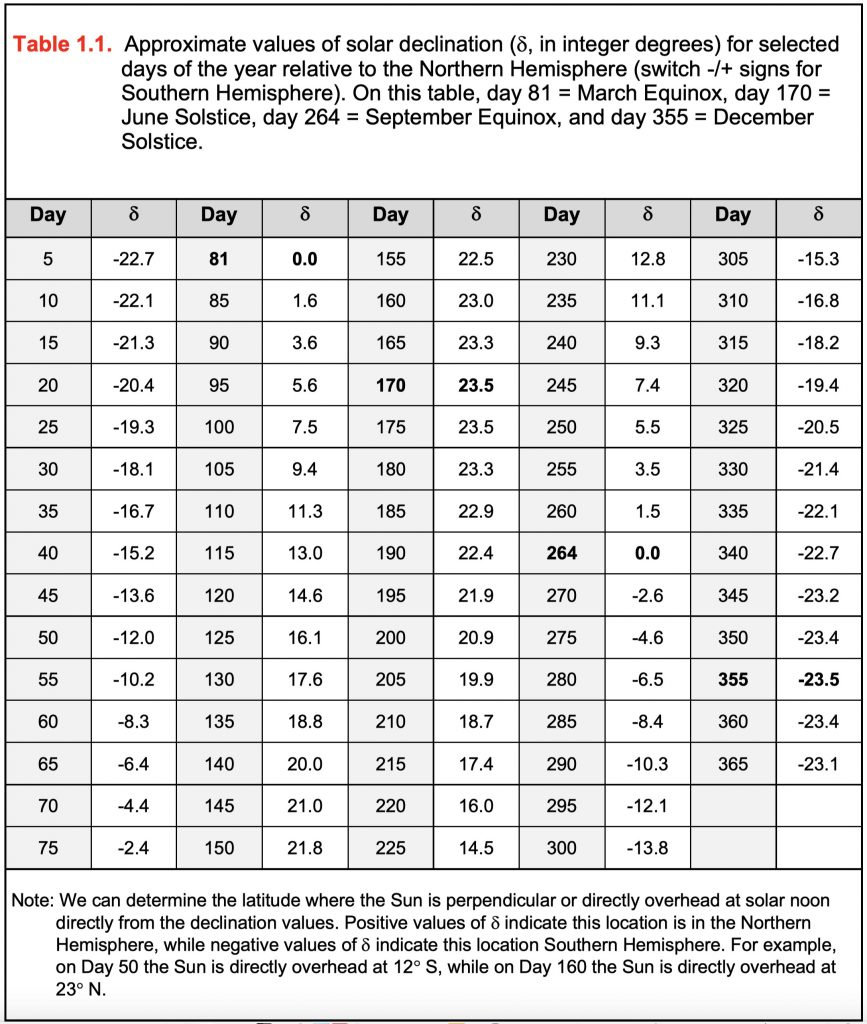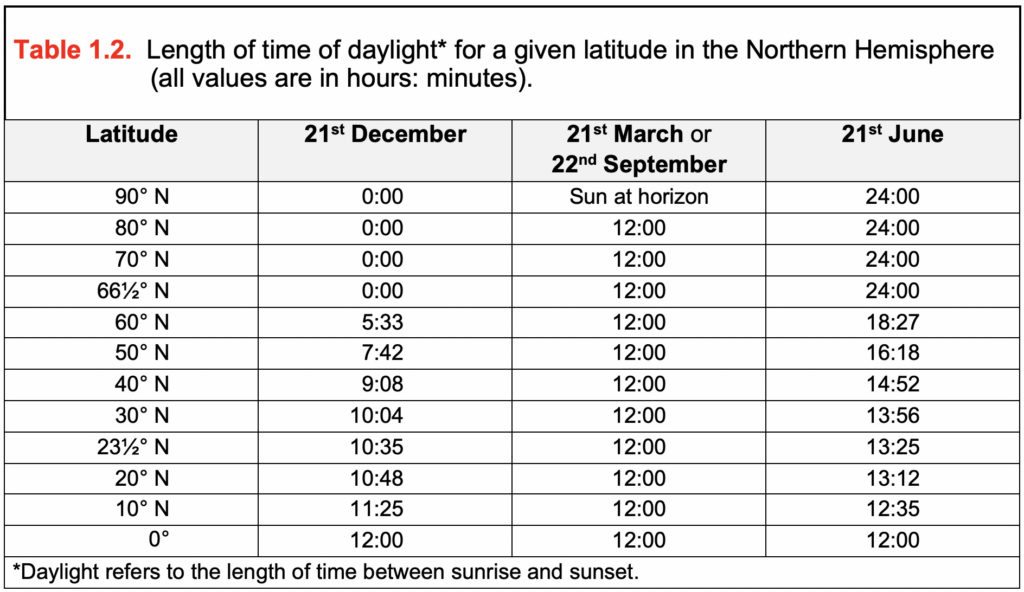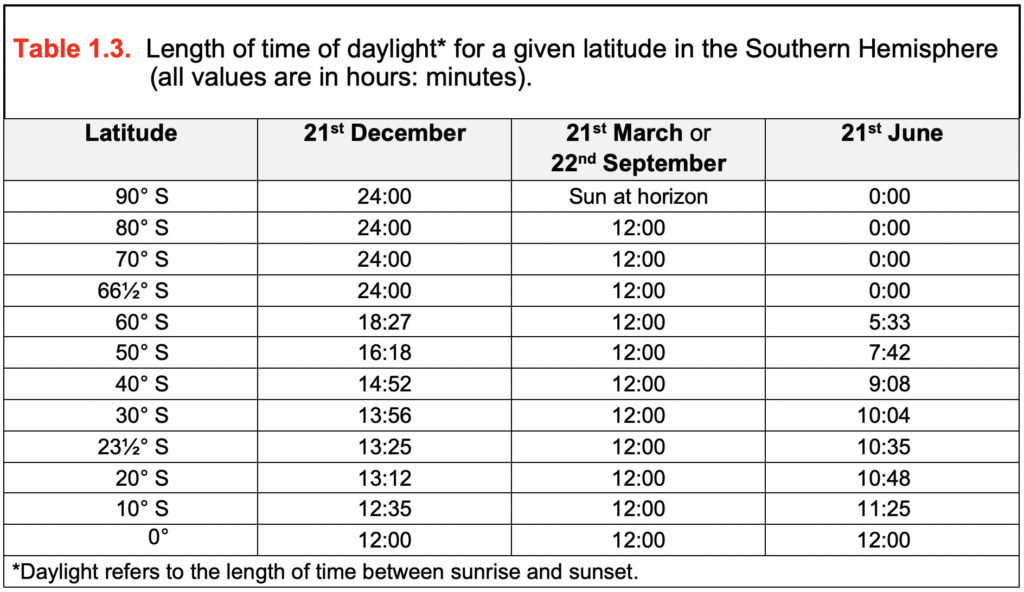4.1) For January 15 (day 15) Sun Angle at Solar Noon = 18.7 degrees; the Relative Intensity of Solar Noon Sun Angle = ______ %; and Day Length = 8 hours and 15 minutes.

4.2) For January 30 (day 30) Sun Angle at Solar Noon = ______ degrees; the Relative Intensity of Solar Noon Sun Angle = ______ %; and Day Length = 8 hours and 58 minutes.

4.3) For March 1 (day 60) Sun Angle at Solar Noon = 31.7 degrees; the Relative Intensity of Solar Noon Sun Angle = ______ %; and Day Length = 10 hours and 26 minutes.

4.4) For March 21 (day 80) Sun Angle at Solar Noon = ______ degrees; the Relative Intensity of Solar Noon Sun Angle = 64%; and Day Length = 12 hours and 0 minutes.

4.5) For April 20 (day 110) Sun Angle at Solar Noon = 51.3 degrees; the Relative Intensity of Solar Noon Sun Angle = ______ %; and Day Length = 13 hours and 46 minutes.

4.6)  For May 20 (day 140) Sun Angle at Solar Noon = ______ degrees; the Relative Intensity of Solar Noon Sun Angle = ______ %; and Day Length = 15 hours and 23 minutes.

4.7) For June 21 (day 140) Sun Angle at Solar Noon = ______ degrees; the Relative Intensity of Solar Noon Sun Angle = ______ %; and Day Length = ______ hours and ______ minutes.

4.8)For August 23 (day 235) Sun Angle at Solar Noon = ______ degrees; the Relative Intensity of Solar Noon Sun Angle = ______ %; and Day Length = 13 hours and 55 minutes.

4.9) For September 22 (day 265) Sun Angle at Solar Noon = ______ degrees; the Relative Intensity of Solar Noon Sun Angle = ______ %; and Day Length = ______ hours and ______ minutes.

4.10)  For October 22 (day 295) Sun Angle at Solar Noon = ______ degrees; the Relative Intensity of Solar Noon Sun Angle = ______ %; and Day Length = 10 hours and 26 minutes.

4.11) For December 22 (day 356) Sun Angle at Solar Noon = ______ degrees; the Relative Intensity of Solar Noon Sun Angle = ______ %; and Day Length = ______ hours and ______ minutes.

4.12) For December 31 (day 365) Sun Angle at Solar Noon = 16.9 degrees; the Relative Intensity of Solar Noon Sun Angle = ______ %; and Day Length = 7 hours and 55 minutes.

4.13) What progression of seasons are we observing for this location from December 31 to June 21?

Winter > Spring > Summer.

Summer > Fall > Winter.

Spring > Summer > Fall.

4.14) Describe what happens to the relative intensity of solar insolation at solar noon from June 21 to December 22.

It increases.

It decreases.

Stays the same.

# QUESTION 5

5.1) During the June Solstice at Solar Noon

The Sun is directly overhead (90 degrees) at ______  degrees ______ (North or South).

The Sun is at 66.5 degrees above the horizon at degrees ______ North and the ______ (name place).

The Sun is at the horizon at ______ degrees ______ (North or South).

5.2) During the December Solstice at Solar Noon

The Sun is directly overhead (90 degrees) at ______ degrees ______ (North or South).

The Sun is at 66.5 degrees above the horizon at ______ degrees South and the ______ (name place).

The Sun is at the horizon at ______ degrees ______ (North or South).

5.3) During the Equinoxes at Solar Noon

The Sun is directly overhead (90 degrees) at the ______ (name place).

The Sun is at 66.5 degrees above the horizon at ______ degrees South and at ______ degrees North.

The Sun is at 10 degrees above the horizon at ______ degrees North and ______ degrees South.

# QUESTION 6

So far in this lab, we have discovered four important facts:

1. The height of the Sun varies on a daily cycle with maximum Sun height occurring at solar noon. These daily cycles are due to Earth rotation.
2. The height of the Sun also varies annually because of changes in the position of the Earth’s axis relative to the Sun.
3. Changes in the position of the Earth’s axis relative to the Sun also influence day lengths, except for locations on the Equator.
4. The potential intensity of the Sun’s insolation is influenced by the angle of incidence.

Using this knowledge and the potential insolation data found in Table 1.4 answer the following questions.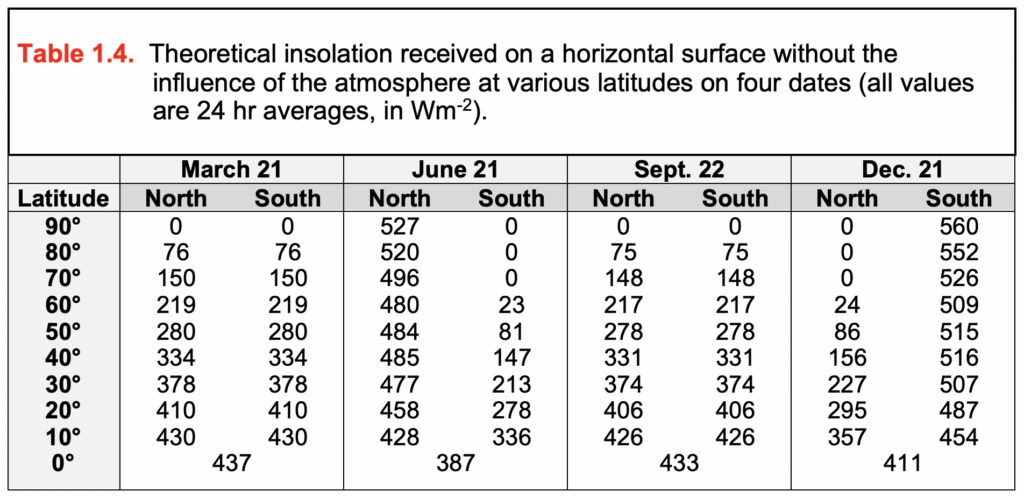6.1) How much insolation is received at 20° North on December 22?

__________  Watts per meter square.

6.2) How much insolation is received at 20° South on June 21?

__________  Watts per meter square.

6.3) What factors account for the difference in insolation received in questions 6.1 and 6.2? Review Figures 1.2 and 1.3 before answering this question. (select one or more correct responses)

The Earth’s elliptical orbit causes our planet to be closer to the Sun around Perihelion.

Differences in day length.

Differences in Sun angles.

6.4) How much insolation is received at 60° North on December 22?

__________  Watts per meter square.

6.5) How much insolation is received at the equator (0°) on December 22?

__________  Watts per meter square.

6.6) What factors account for the difference in insolation received in questions 6.4 and 6.5? Review Figures 1.2 and 1.3 before answering this question. (select one or more correct responses)

The Earth’s elliptical orbit causes our planet to be closer to the Sun around Perihelion.

Differences in day length.

Differences in Sun angles.

6.7) How much insolation is received at the equator (0°) on September 23?

__________  Watts per meter square.

6.8) How much insolation is received at 60° North on September 23?

__________  Watts per meter square.

6.9) What factors account for the difference in insolation received in questions 6.7 and 6.8? Review Figures 1.2 and 1.3 before answering this question. (select one or more correct responses)

The Earth’s elliptical orbit causes our planet to be closer to the Sun around Perihelion.

Differences in day length.

Differences in Sun angles.

# IMAGE CREDITS

Figure 1.2: Image Copyright Michael Pidwirny.

Figure 1.3: Image Copyright Michael Pidwirny.

Figure 1.4: Image Copyright Michael Pidwirny.

Figure 1.5: Image Copyright Michael Pidwirny.

Figure 1.7: Image Copyright Michael Pidwirny.

Figure 1.8: Image Copyright Michael Pidwirny.

Figure 1.9: Image Copyright Michael Pidwirny.

Figure 1.10: Image Copyright Michael Pidwirny.

Figure 1.11: Image Copyright Michael Pidwirny.

# FIGURES AND TABLES – PDF FILES

Lab 1 Tables 1.1, 1.2, and 1.3

Lab 1 Table 1.4

Lab 1 Figures 1.9, 1.10, and 1.11

# MICROSOFT EXCEL DATA FILES